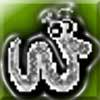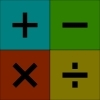#### You may also like### Worms

Place this "worm" on the 100 square and find the total of the four squares it covers. Keeping its head in the same place, what other totals can you make?### Which Scripts?

There are six numbers written in five different scripts. Can you sort out which is which?### Highest and Lowest

Put operations signs between the numbers 3 4 5 6 to make the highest possible number and lowest possible number.

# The Magic Number and the Hepta-tree

##### Age 7 to 11Challenge Level

We received many solutions to this problem, although not so many of you explained exactly how you arrived at your answer. Remember, we're always on the look out for your reasoning too.

Elijah let us know his estimates of the number on each ball:

$7\%$ of $614$ is ~$50$
$13\%$ of $330$ is ~$41$
$17\%$ of $253$ is ~$40$
$19\%$ of $226$ is ~$40$
$23\%$ of $187$ is ~$43$
$61\%$ of $71$ is ~$42$
$77\%$ of $56$ is ~$36$

You didn't say how you made these estimations, Elijah. It would be interesting to know ...

'Team Sean', which consisted of Sean, Liz, Briana, Alysha, Amy, Amelia, Chris, Ben, Charlie, Kaden, Matt, Josh, Kate, Stacey, Roy, Mitchell, Dylan, Ed, Emma and Grace gave a detailed explanation of their method for finding the magic number:

Step $1$ -
We turned the percentages into fractions and divided the top by the bottom, then we multiplied that answer by the number in the ball. (Alysha)
Example - (Briana) $\frac{23}{100} \times 187 = 43.01$

Step $2$ -
We found the biggest and smallest answers and subtracted the small one from the bigger one. (Dylan)
(Liz) $43.31-42.9 = 0.41$

Step $3$ -
You multiply the answer by $244$ (Josh)
(Sean) $0.41 \times 244 = 100.04$

Step $4$ -
On a number line, from $100.04$, you need $96$ more hundredths to get to $101$ but you only need to go back $4$ hundredths to get to $100$.
Therefore the magic number is $100$ because $100.04$ rounds down to $100$. (Alysha, Charlie and Matt)

We also received good solutions from Adam and Chris who both go to Orchard Primary School, and Callum and Eddie from Stourport Primary. Lucy used a spreadsheet to calculate the percentages rather than a calculator, which works equally well. Well done all of you!